# SSC CGL EXAMS 2018 | Reasoning Ability Practice Questions (Day-14)

Dear Aspirants, Here we have given the Important SSC Exam 2018 Practice Test Papers. Candidates those who are preparing for SSC 2018 can practice these questions to get more confidence to Crack SSC 2018 Examination.

[WpProQuiz 4600]

1) If S1T means “S is mother of T”, “S3T” means “S is Son of T” and “S5T” means “S is Daughter of T, then how is E is related to B in B5S1E3D?

a) Son in law

b) Daughter

c) Grand Son

d) Can’t be determine

2) In the following question, from the given alternatives words, select the word which can be formed using the letters of the given word.

NOTORIOUS

a) Tore

b) Rose

c) Tour

d) Noun

3) In a certain code, “TAB” is coded as “5” and “HUB” is coded as “4”. How is “INK” coded in that language?

a) 4

b) 3

c) 2

d) 7

4) In the following question, select the related letters from the given responses

PACE : QBDF : : ROLE : ?

a) SPME

b) SKPE

c) SMPF

d) EMPS

5) Arrange the given words in the sequences in which they occur in dictionary.

(i) Rebuke

(ii) Ravage

(iii) Rivalry

(iv) Renewable

(v) Robust

a) (iv), (i), (iii), (v), (ii)

b) (ii), (iv), (i), (v), (iii)

c) (ii), (i), (iv), (ii), (v)

d) (iii), (iv), (i), (v), (ii)

6) A series is given with one term missing. Select the correct alternate from the given ones that will complete the series.

BEHK, DGJM, GJMP,  ?

a) KNQT

b) EGJN

c) HJMQ

d) KMPT

7) In the following question, correct the equation by interchanging two signs

7 ÷ 2 + 120 × 5 – 12 = 26

a) ÷ and ×

b) + and –

c) ÷ and +

d) – and ÷

8) If 2*5%3 = 1000 and 5*4%2 = 400, then 5*3%3 =?

a) 225

b) 3375

c) 362

d) 140

9) In the following question, select the number which can be placed at the sign of question mark (?) from the given alternative.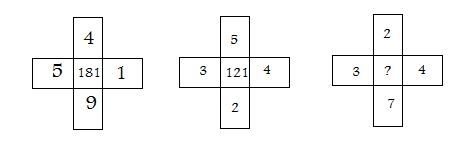a) 170

b) 144

c) 169

d) 130

10) How many rectangles are there in the given figure?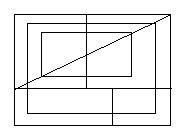a) 18

b) 16

c) 10

d) 17

S1T – S is mother of T

S3T – S is Son of T

S5T – S is Daughter of T

In the relationship of B5S1E3D is

Alphabets are coded as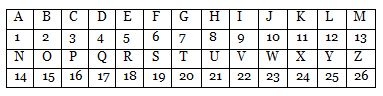TAB = 20 + 1 + 2 = 23 = 5(2 + 3)

HUB = 8 + 21 + 2 = 31 = 4 (3 + 1)

INK = 9 + 14 + 11 = 34 = 7 (3 + 4)The correct order is

(ii). Ravage

(i). Rebuke

(iv). Renewable

(iii). Rivalry

(v). Robust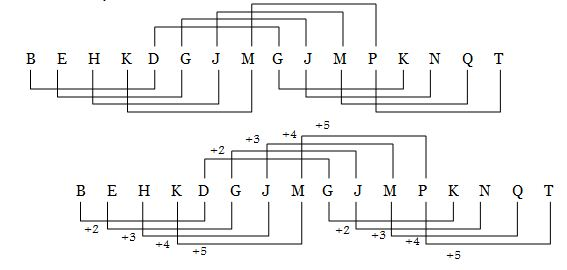7 ÷ 2 + 120 × 5 – 70 = 42

By changing ÷ and ×, equation will be

7 × 2 + (120 ÷ 5) – 70 = 26

14 + 24 – 12 = 26

2*5%3 = 1000 => 2 × 5^3 = 103 = 1000

5*4%2 = 400 => 5 × 4^3 = 202 = 400

5*3%3 = 5 × 3^3 = 153 = 3375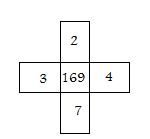5*4*1*9 = 180 + 1 = 181

5*3*4*2 = 120 +1 = 121

3*2*3*7 = 168 + 1 = 169

There are 17 rectangles.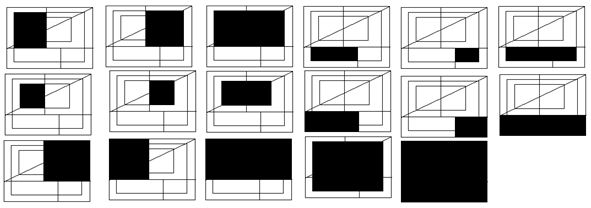*********************************